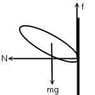# Circular motion- slipping problem

• crossfacer

#### crossfacer

Circular motion-----"slipping" problem

## Homework Statement

The figure attached shows a man riding a motorcycle on the rough wall of a cylindrical room. He is performing uniform circular motion and does not slide down along the wall.

If the man rides the motorcycle and performs circular motion along the rough wall without slipping, prove that the following condition must hold:
1 <= (u*v^2) / rg​
where u is the coefficient of static friction, v is the speed of the motorcycle, r is the radius of the circle and g is the acceleration due to gravity.

## The Attempt at a Solution

If the motorcycle does not slip,
N >= mv^2 / r------------(i)​
Consider vertical motion:
f - mg = 0
f = mg
uN = mg
N = mg / u-------------(ii)​
Substitute (ii) to (i):
mg / u >= (mv^2) / r
1 >= (uv^2) / rg​

In the solution, the answer uses:
mg <= f-------(I)
and N = (mv^2) / r-----------(II)​
But why (I) is correct when the motorcycle does not slip? If mg > f, will the motorcycle go upward? And what's wrong with my answer? Thank you so much!

#### Attachments

•forcediagram.jpg
2.3 KB · Views: 481
Last edited:
while waiting for the pic to approve, and being able to confirm your FBD:

I) Obtains from the condition that the frictional force be at least equal to the gravitation force, ie N*u*m>=m*g , and since the normal force is equal to the centripetal force, mv^2/R, then (II).
subbing gives, (mv^2/R)*u>=g, divide by gee, and QED.

There is no upward force here, so at best the bike won't fall. Your problem was assuming that the inequality was with the normal force and not the gravitational. Its the other way around. That help?

I can't see your picture (still waiting for approval) but as denverdoc said your equations (i) and (ii) are not quite right:

(i) should be an equality, for circular motion: N = mv^2/r
(ii) is should be an inequality saying the maximum possible friction force must be greater than the weight: uN > mg. Of course the actual friction force is less than uN and equals the weight.

These two equations are not a full description of the problem. The weight and the centripetal force act at the CG of the bike. The reaction forces (normal and friction) act at the point of contact of the bike and the wall. The equal and opposite forces (two horizontal, two vertical) produce two moments.

If the biker leans "upwards" by the right amount, and steers "straight ahead", these two moments can be equal and he will ride in a circle. If he leans further "upwards" he can climb higher up the wall. If he tries to ride "upright" (i.e. in the horizontal plane) the bike will topple over downwards, for any values of the speed and friction coefficient are.

OT: How do the gyroscopic "forces" from the rapidly spinning wheels enter into this AZ?

OT: How do the gyroscopic "forces" from the rapidly spinning wheels enter into this AZ?

My guess (without doing any serious calcs) would be "not much". When the bike is leaning "upwards", the axis of rotation of the wheels is precessing one rev round a vertical axis for one rev round the wall. I think that would be a slow rate compared with the rate of turning the handlebars for steering corrections etc.

If the bike goes faster, the couple from the weight + friction forces remains constant. The centripetal force and normal reaction increase proportional to speed^2, so the angle of "lean" will be roughly proportional to 1/speed^2 (assuming small angles) to balance the two couples. The rotation speed of the wheels is proportional to speed, so the overall effect of gyro would be proportional to 1/speed.

I would think the effect of increased "g force" on the rider (and bike) is more significant, since it might increase the effective weight of the rider and bike by a factor of 2 or 3. Any "sideways wobbles" are going to take effect faster (proportional to the g force) because of that - the weight is increased, but the inertia is unchanged.

Even more OT: I wonder of Evil Knevil and his colleagues use bikes with modified steering geometry - e.g. with a bigger "castor angle" effect on the front wheel so they are more stable in a straight line than normal bikes...

Last edited:
http://www.globeofdeath.info/inside-pics.html [Broken]

These are 125cc trail bikes sans brakes.Last edited by a moderator: